## LuGre friction model in MATLAB

version 4.0 (1.5 MB) by
A full reconstruction of the paper: A new model for control systems with friction

Updated 25 Jul 2021

From GitHubReconstruction of the paper: A new model for control systems with friction
By: Canudas de Wit et al., 1995, IEEE Transactions on Automatic Control

I am trying to reconstruct as many figures as possible from the paper above.

There are 2 m-files:

1. demo2.m

This is the second attempt at reconstructing the paper. Here, I use the built-in MATLAB solver: ode23s, which is designed for a stiff system. Thus, it takes less time to complete the simulation. In order to use MATLAB built-in solver, the problem must be first formalized. Plese see the PDF file here.

1. demo3.m

This is an additional simulation to demonstrate the friction observer. This is not shown in the paper. Basically, this is a numerical simulation of section V.B, for position control with a unit-step input.

1. demo4.m

This shows optimally tuned PI and velocity gain without the friction observer. The performance is superior to the friction observer with poorly tuned PI and velocity gains.

Not shown in the paper. Run demo2.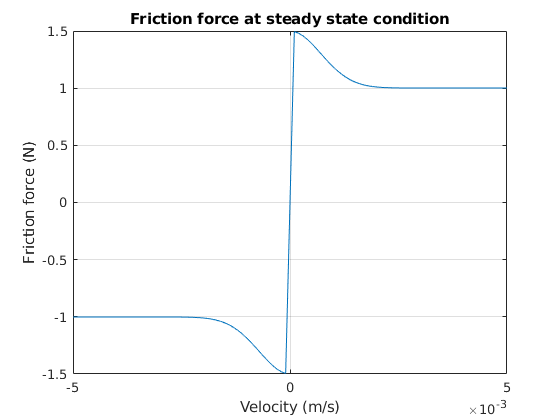Not shown in the paper. Run demo2.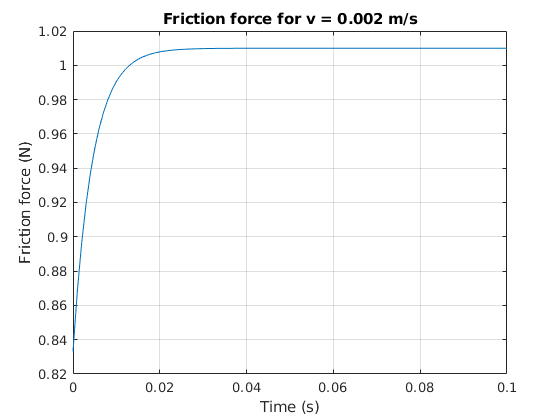Fig. 3 of the paper. Run demo2.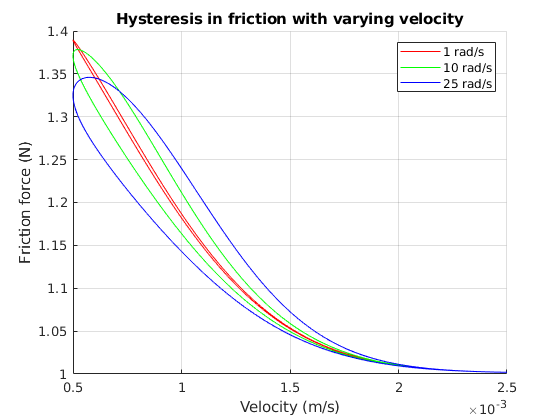Fig. 2 of the paper. Run demo2.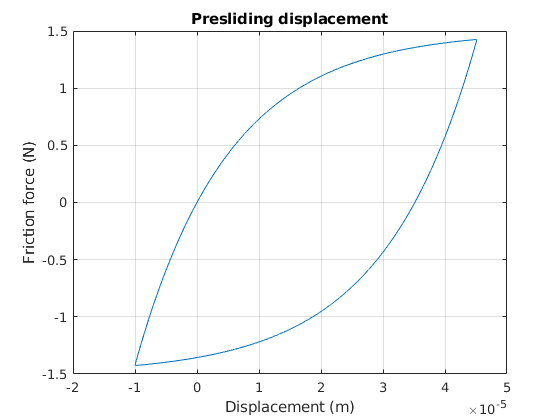Fig. 6 of the paper. Run demo2.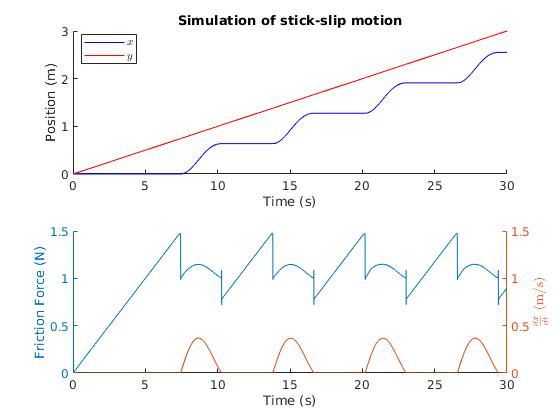Fig. 4 of the paper. Run demo2.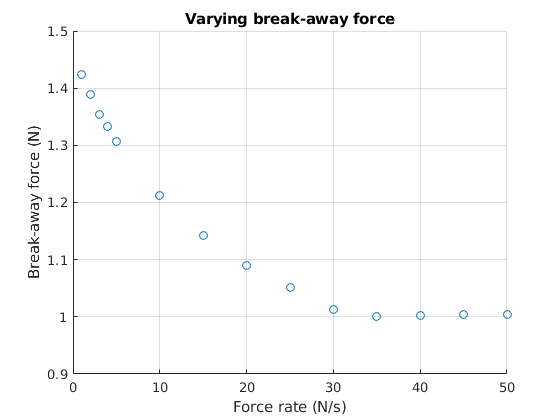Fig. 8 of the paper. Run demo2.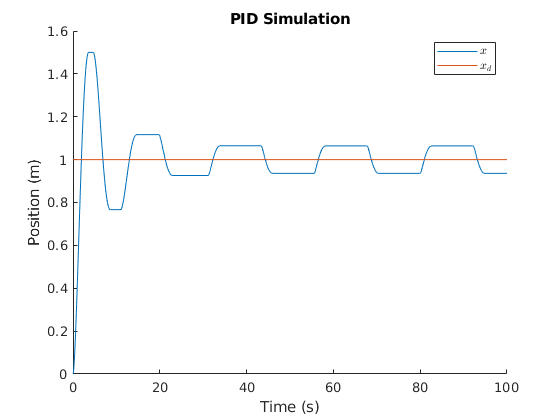Not shown in the paper. Run demo3.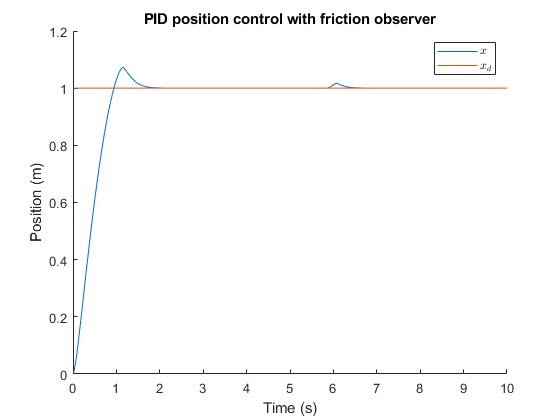Note that if the PI and velocity gains are optimally tuned, then the performance is better than the friction observer. Rise time is smaller and overshoot is smaller. This model is generally easier to tune. Run demo4.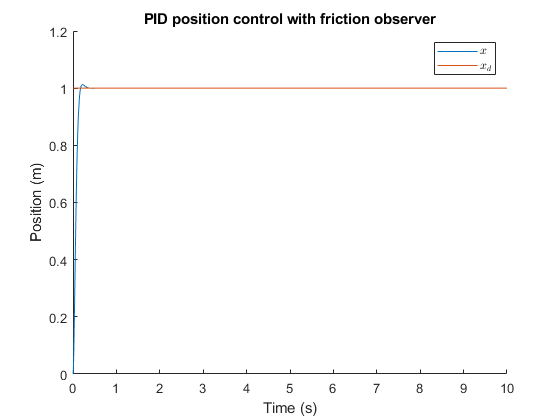### Cite As

Auralius Manurung (2021). LuGre friction model in MATLAB (https://github.com/auralius/LuGre/releases/tag/4.0), GitHub. Retrieved .

##### MATLAB Release Compatibility
Created with R2018b
Compatible with any release
##### Platform Compatibility
Windows macOS Linux